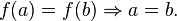# Injection

A function is injective (one-to-one) if every possible element of the codomain is mapped to by at most one argument. Equivalently, a function is injective if it maps distinct arguments to distinct images. An injective function is an injection. The formal definition is the following.

The functionis injective iff for all, we have• A function f : AB is injective if and only if A is empty or f is left-invertible; that is, there is a function g : f(A) → A such that g o f = identity function on A. Here f(A) is the image of f.
• Since every function is surjective when its codomain is restricted to its image, every injection induces a bijection onto its image. More precisely, every injection f : AB can be factored as a bijection followed by an inclusion as follows. Let fR : Af(A) be f with codomain restricted to its image, and let i : f(A) → B be the inclusion map from f(A) into B. Then f = i o fR. A dual factorisation is given for surjections below.
• The composition of two injections is again an injection, but if g o f is injective, then it can only be concluded that f is injective. See the figure at right.
• Every embedding is injective.

Posted on October 5, 2011, in education and tagged , , , , , , , . Bookmark the permalink. 3 Comments.

1.irfan handi